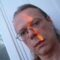# The Right is Right, But the Left is More Right: A Mathematical Proof

We’ll start this proof with a simple relation.
Right angle = 90 degree angle
Therefore, Right =90 degrees.

Now we’ve all heard of “doing a 180” which is to do the opposite. This comes from the mathematics which means to change by 180 degrees.
So then Left = 180 degree change from right

But we don’t mean to take away from what is Right. And of course we don’t want this to end with a negative – I’d hate to be such a downer.
Then Left = Right + 180 degrees = 90 degrees + 180 degrees = 270 degrees
Therefore Left = 3 x Right = more Right

The astute among you might notice we could keep going in a circle and define Right as being 180 degrees more than Left or 5 x Right. Then Left could say it’s 7 x Right, etc. But this is both dizzying and roundabout. And we don’t want to add these vicious cycles to our simple relation.

Therefore, Right is Right, But Left is more Right.

Update 2013/04/16 :
Then there is the non Euclidean geometry proof. Say you lie down on your back both arms extended in opposite directions. Outline this position. Follow that right hand out further than it goes, in a straight line. Eventually you will have rounded the earth and come back to your outline – on your left side.

Going further than your left side is both dizzying and roundabout as before. So your left is the most right in non Euclidean geometry.